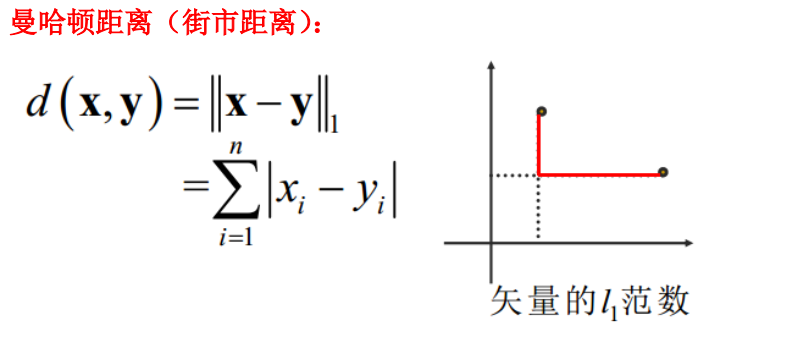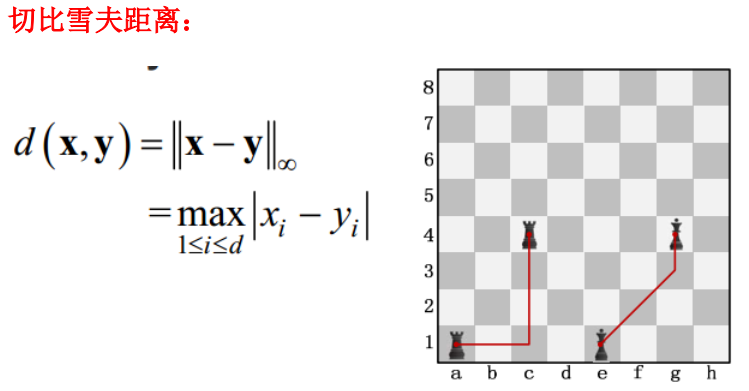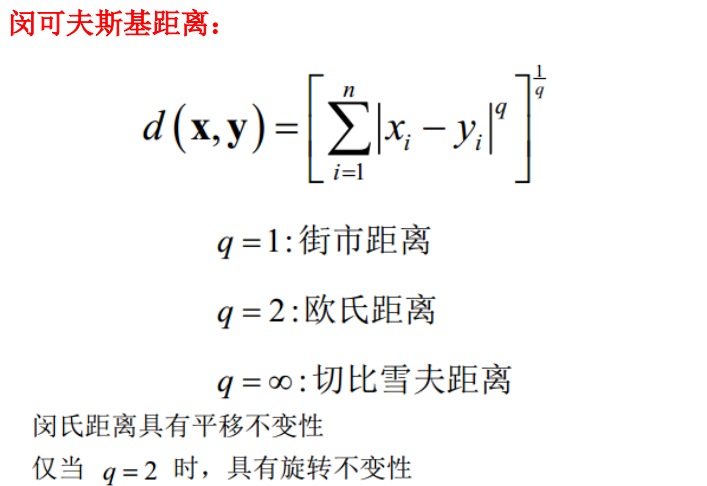python实现常用的相似度计算方法

369 篇文章 4 订阅

相似度计算是很多具体的应用了里面都会使用到的一些东西，我们学过的有很多相似度计算的方法，最初的相似度计算是为了表征向量的重合程度的，在这里最经典的就是余弦相似度了，当然使用正弦或者是正切等等三角函数也都是可以的，只不过余弦使用的更广泛一些所以提到三角函数计算向量相似度的时候大家往往都会使用余弦来作为相似度的计算工具。

可能最开始会觉得相似度计算没有什么，因为现在已经有很多应用于了实践的相似度计算方法，但是你可能不太了解很多任务里面的核心工作就是在进行相似度计算。比如文本情感分析、文本语义理解、商场系统里面的个性化推荐等等，相似度计算可以独立进行也可以是作为某一项具体任务里面的一部分进行，不同的业务场景里面会需要用到不同的相似度计算策略。比如：给定两个字符串，想要计算两个字符串之间的相似度，那么可能最好的相似度计算方法就会是字符串的编辑距离了；给定两个一维的向量数据，想要计算这一对向量之间的相似度，那么可能最直接的相似度计算策略就会是余弦相似度了；给定两个商品，以及商品各自的很多属性，或者是给定两个人以及每个人各自的性格、兴趣、爱好等等，想要来计算商品或者是人物之间的相似度那么可能最好的相似度计算方法就会是杰卡德相似系数了。因为Jaccard系数主要用于计算符号度量或布尔值度量的个体间的相似度，因为个体的特征属性都是由符号度量或者布尔值标识，因此无法衡量差异具 体值的大小，只能获得“是否相同”这个结果，所以Jaccard系数只关心个体间共同具有的特征是否一致这个问题。

类似上面的实例还会有很多，简单列举这些知识想说明：在不同的人物场景里面，为了完成特征的计算任务，我们选择的计算策略和方法往往都是不同的。

除此之外，还有距离计算方法可以用来计算相似度，比如：欧式距离、闵可夫斯基距离、切比雪夫距离等等，都是广泛用来计算相似度的工具，本文简单整理了一些统计学中常用的距离计算方法如下：

1、欧几里得距离（Eucledian Distance）2、曼哈顿距离（Manhattan Distance）3.切比雪夫距离4、明可夫斯基距离（Minkowski distance）处理上面列举的一些距离方法之外还有一种经典的距离计算方法叫做：编辑距离。编辑距离经常用于计算字符串之间的差异或者说是相似度，感兴趣的也可以去查一下相关的资料，这里就不再多解释了。

除此之外，还有统计学中的三大相关性系数：皮尔森系数、斯皮尔曼系数和肯德尔系数。这些都是可以直接拿来计算向量之间的相似度的。另外还可以使用集合来计算相似度，杰卡德系数就是这一方面的实例，两个集合的交集除以两个集合的并集来作为两个集合的相似度。

本文对常用的一些相似度计算方法进行总结实现，具体内容如下：

#!usr/bin/env python
#encoding:utf-8
from __future__ import division

'''
__Author__:沂水寒城

'''

import math
import numpy as np
from scipy.stats import pearsonr,spearmanr,kendalltau

import sys
sys.setdefaultencoding('utf-8')

def pearsonrSim(x,y):
'''
皮尔森相似度
'''
return pearsonr(x,y)

def spearmanrSim(x,y):
'''
斯皮尔曼相似度
'''
return spearmanr(x,y)

def kendalltauSim(x,y):
'''
肯德尔相似度
'''
return kendalltau(x,y)

def cosSim(x,y):
'''
余弦相似度计算方法
'''
tmp=sum(a*b for a,b in zip(x,y))
non=np.linalg.norm(x)*np.linalg.norm(y)
return round(tmp/float(non),3)

def eculidDisSim(x,y):
'''
欧几里得相似度计算方法
'''
return math.sqrt(sum(pow(a-b,2) for a,b in zip(x,y)))

def manhattanDisSim(x,y):
'''
曼哈顿距离计算方法
'''
return sum(abs(a-b) for a,b in zip(x,y))

def minkowskiDisSim(x,y,p):
'''
明可夫斯基距离计算方法
'''
sumvalue=sum(pow(abs(a-b),p) for a,b in zip(x,y))
tmp=1/float(p)
return round(sumvalue**tmp,3)

def MahalanobisDisSim(x,y):
'''
马氏距离计算方法
'''
npvec1,npvec2=np.array(x),np.array(y)
npvec=np.array([npvec1, npvec2])
sub=npvec.T-npvec.T
inv_sub=np.linalg.inv(np.cov(npvec1, npvec2))
return math.sqrt(np.dot(inv_sub, sub).dot(sub.T))

def levenshteinDisSim(x,y):
'''
字符串编辑距离、相似度计算方法
'''
res=Levenshtein.distance(x,y)
similarity=1-(res/max(len(x), len(y)))
return similarity

def jaccardDisSim(x,y):
'''
杰卡德相似度计算
'''
res=len(set.intersection(*[set(x),set(y)]))
union_cardinality=len(set.union(*[set(x),set(y)]))
return res/float(union_cardinality)

if __name__=='__main__':
x=[1,2,3,4,5]
y=[2,4,0,8,9]
print 'pearsonrSim:',pearsonrSim(x,y)
print 'spearmanrSim:',spearmanrSim(x,y)
print 'kendalltauSim:',kendalltauSim(x,y)
print 'cosSim:',cosSim(x,y)
print 'eculidDisSim:',eculidDisSim(x,y)
print 'manhattanDisSim:',manhattanDisSim(x,y)
print 'minkowskiDisSim:',minkowskiDisSim(x,y,2)
print 'MahalanobisDisSim:',MahalanobisDisSim(x,y)
print 'jaccardDisSim:',jaccardDisSim(x,y)

结果如下：

pearsonrSim: 0.7397954428741078
spearmanrSim: 0.7
kendalltauSim: 0.6
cosSim: 0.913
eculidDisSim: 6.78232998313
manhattanDisSim: 14
minkowskiDisSim: 6.782
MahalanobisDisSim: 0.637157868392
jaccardDisSim: 0.25

记录一下！

09-23
11-211万+

01-29355
01-132万+
10-181785
12-061100
01-281028
01-113226
08-301万+
06-012万+
12-041351
11-172万+
08-16372
07-168447
01-184万+
10-172364
11-20133
12-09166
03-128571
11-19854Together_CZ

¥2 ¥4 ¥6 ¥10 ¥20余额支付 (余额：-- )扫码支付获取中扫码支付点击重新获取扫码支付1.余额是钱包充值的虚拟货币，按照1:1的比例进行支付金额的抵扣。
2.余额无法直接购买下载，可以购买VIP、C币套餐、付费专栏及课程。余额充值